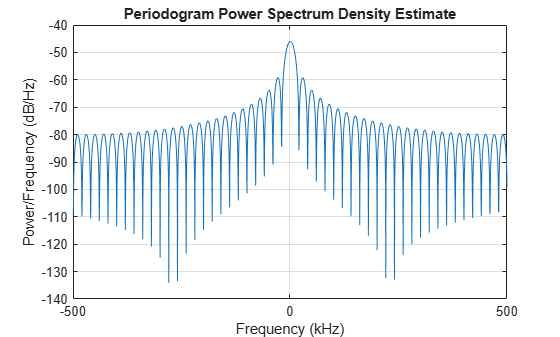step

System object: phased.StretchProcessor
Package: phased

Perform stretch processing for linear FM waveform

Syntax

Y = step(H,X)
Y = step(H,X,PRF)

Description

Note

Starting in R2016b, instead of using the step method to perform the operation defined by the System object™, you can call the object with arguments, as if it were a function. For example, y = step(obj,x) and y = obj(x) perform equivalent operations.

Y = step(H,X) applies stretch processing along the first dimension of X. Each column of X represents one receiving pulse.

Y = step(H,X,PRF) uses PRF as the pulse repetition frequency. This syntax is available when the PRFSource property is 'Input port'.

Input Arguments

 H Stretch processor object. X Input signal matrix. Each column represents one received pulse.The size of the first dimension of the input matrix can vary to simulate a changing signal length. A size change can occur, for example, in the case of a pulse waveform with variable pulse repetition frequency. PRF Pulse repetition frequency specified as a positive scalar. To enable this argument, set the PRFSource property to 'Input port'. Units are in Hertz.

Output Arguments

 Y Result of stretch processing. The dimensions of Y match the dimensions of X.

Examples

expand all

Use stretch processing to locate a target at a range of 4950 m.

Simulate the signal.

waveform = phased.LinearFMWaveform;
x = waveform();
c = physconst('LightSpeed');
rng = 4950.0;
num_samples = round(rng/(c/(2*waveform.SampleRate)));
x = circshift(x,num_samples);

Perform stretch processing.

stretchproc = getStretchProcessor(waveform,5000,200,c);
y = stretchproc(x);

Plot the spectrum of the resulting signal.

[Pxx,F] = periodogram(y,[],2048,stretchproc.SampleRate,'centered');
plot(F/1000,10*log10(Pxx))
grid
xlabel('Frequency (kHz)')
ylabel('Power/Frequency (dB/Hz)')
title('Periodogram Power Spectrum Density Estimate')Detect the range.

[~,rngidx] = findpeaks(pow2db(Pxx/max(Pxx)),'MinPeakHeight',-5);
rngfreq = F(rngidx);
rng = stretchfreq2rng(rngfreq,stretchproc.SweepSlope,stretchproc.ReferenceRange,c)
rng = 4.9634e+03